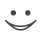Zu dieser Karteikarte gibt es einen kompletten Satz an Karteikarten. Kostenlos!

238
19. If e is a random sample from a standard normal distribution, which of the following is the change in a Wiener process in time dt .
A.e times the square root of dt
B.e times dt
C.dt times the square root of e
D.The square root of e times the square root of dt